# Martynov Vompe

Jump to: navigation, search

The Martynov-Vompe closure relation   is given by: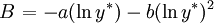$\left.B\right.=-a(\ln y^*) - b(\ln y^*)^2$

where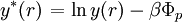$\left.y^*(r)\right. = \ln y(r) - \beta \Phi_p$

where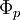$\Phi_p$ is the perturbative part of the pair potential (Note: in the Weeks-Chandler-Andersen separation for the Lennard-Jones system, the `perturbative part' is the attractive part). Martynov and Vompe have used the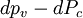$dp_v-dP_c$ and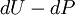$dU-dP$ thermodynamic consistencies in constructing their closures .